Next: Feature selection Up: Properties of Naive Bayes Previous: Properties of Naive Bayes   Contents   Index

## A variant of the multinomial model

An alternative formalization of the represents each document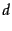as an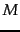-dimensional vector of counts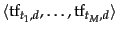where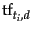is the term frequency of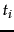in.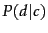is then computed as follows (cf. Equation 99, page 12.2.1 );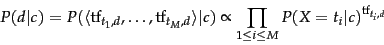(129)

Note that we have omitted the multinomial factor. See Equation 99 (page 99 ).

Equation 129 is equivalent to the sequence model in Equation 113 as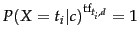for terms that do not occur in(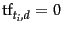) and a term that occurs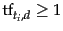times will contributefactors both in Equation 113 and in Equation 129.

Exercises.

• Which of the documents in Table 13.5 have identical and different bag of words representations for (i) the Bernoulli model (ii) the multinomial model? If there are differences, describe them.

• The rationale for the positional independence assumption is that there is no useful information in the fact that a term occurs in position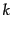of a document. Find exceptions. Consider formulaic documents with a fixed document structure.

• Table 13.3 gives Bernoulli and multinomial estimates for the word the. Explain the difference.Next: Feature selection Up: Properties of Naive Bayes Previous: Properties of Naive Bayes   Contents   Index